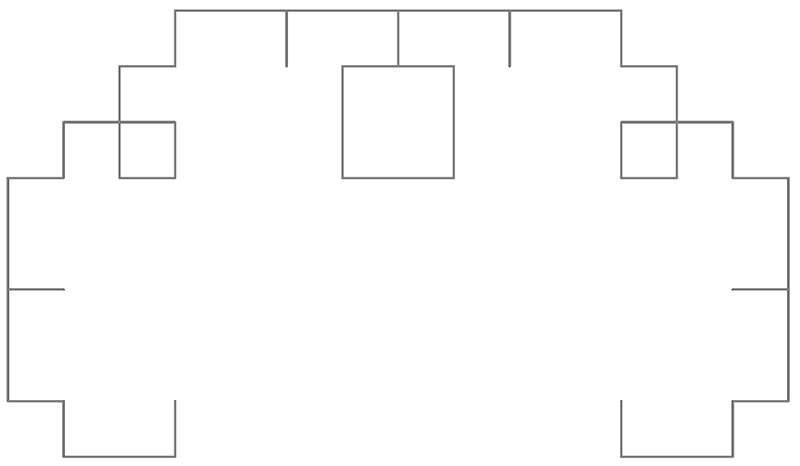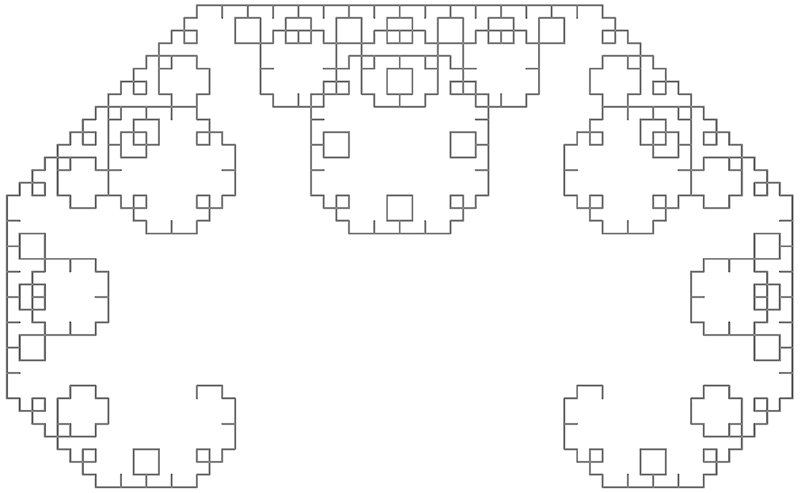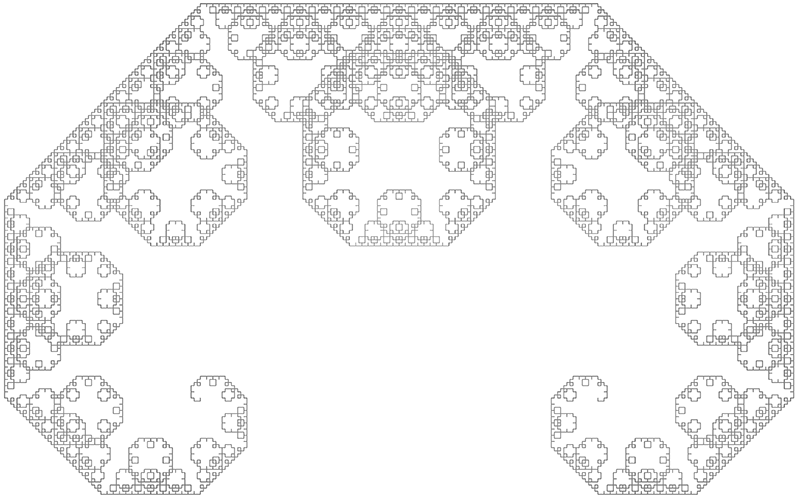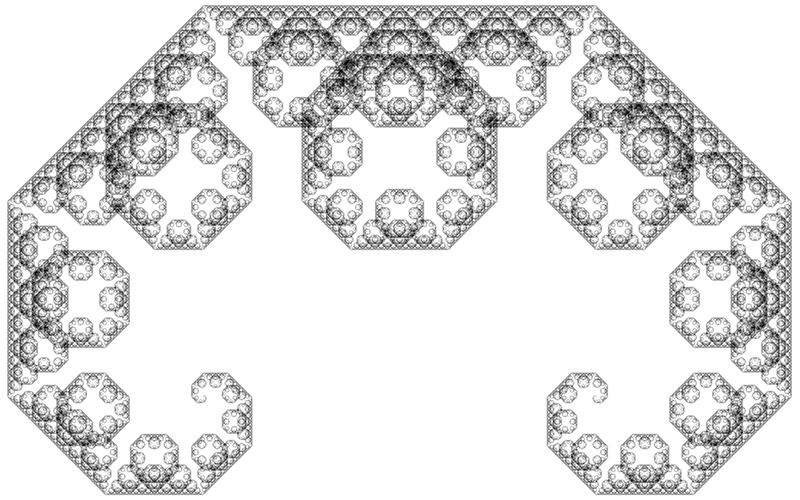# Lévy Curve

Also known on the "C curve" when drawn on its side
Written by Paul Bourke
February 2018

First reported by Ernesto Cesàro in 1906 and Georg Faber in 1910 but named after Paul Lévy who studied its properties in more depth. It can be formulated as both a L-System and IFS (Iterated Function System, both as provided below.

L-System formulation
axiom = F
F -> -F++F-
angle = 456th iteration, 316 line segments10th iteration, 5116 line segments14th iteration, 81916 line segments

Iterated Function System formulation1000 million points. Bounds: -0.5 <= x <= 0.5, and -0.5 <= y <= 0.0125 where z = x+iy